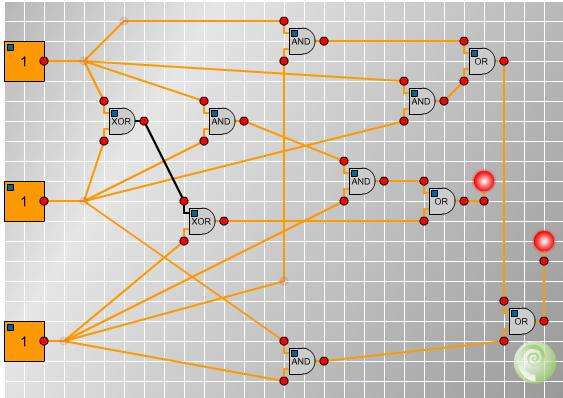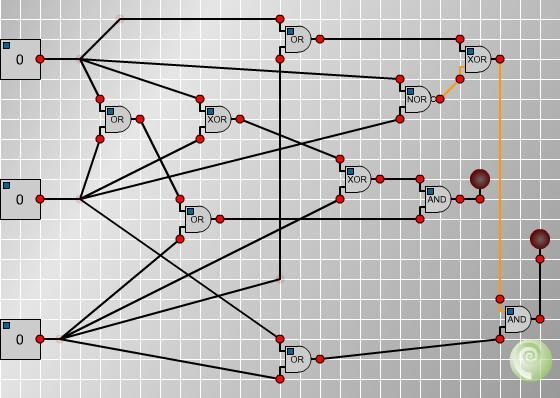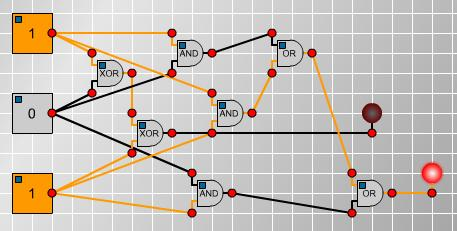You may also likeOverarch 2

Bricks are 20cm long and 10cm high. How high could an arch be built without mortar on a flat horizontal surface, to overhang by 1 metre? How big an overhang is it possible to make like this?Stonehenge

Explain why, when moving heavy objects on rollers, the object moves twice as fast as the rollers. Try a similar experiment yourself.Maximum Flow

Given the graph of a supply network and the maximum capacity for flow in each section find the maximum flow across the network.

Age 16 to 18 Challenge Level:

Two solvers cracked the circuit: Ruth from Manchester High School for Girls and Patrick from Woodbridge School. Rather nicely, they found different solutions to the problem. This raises the question: are there other solutions?

Ruth : I came up with the solution by considering the cases when each bulb lights. The right bulb lights when there are at least 2 switches on. This means that at least one pair of switches must be both on, so if you put each pair into an 'AND' gate to get a pair with both on then combine these with two 'OR' gates so that if any one of the 'AND' gates are lit, the bulb is lit, the bulb then lights when at least two are lit. The left bulb lights when either one or three switches are on. All three switches being on is quite easy: put the first two into an 'AND' gate and combine this with the third with another 'AND' gate. To get when exactly one is lit use two 'XOR' gates connected like the 'AND' gates. connect these two with an 'OR' gate into the left bulb.

Patrick correctly realised that this circuit is a 'binary adder' :

Patrick : The logic gates represent a simple counting device for binary - each switch counts for 1, the left light is the 1s column in binary, and the right light is the 2s column in binary. Thus, if no switch is on, the result is 0. If any one switch is on, the result is 1. If any two switches are on, the result is 2, which in binary is 10. If all three switches are on, the result is 3, which in binary is 11.

Ruth's and Patrick's solution circuits are :Rather nicely, Ruth also noticed that a simpler circuit did the trick, and that some of the gates were redundant. This was an excellent piece of logical analysis!

"Another look at the circuit shows that we have 3 unecessary gates: the 'AND' gates to give when 3 are on, and also the 'OR' to combine this with the result of the 'XOR's, can be removed without changing the output, as when all 3 are on the first 'XOR' is off, so the second is on, as follows: